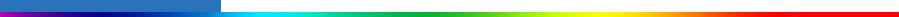Working Group I: The Scientific BasisGet Javascript Other reports in this collection

 Appendix 12.2: Three Approaches to Optimal Detection Optimal detection studies come in several variants depending upon how the time evolution of signal amplitude and structure is treated. Fixed pattern studies (Hegerl et al., 1996, 1997, 2000a; Berliner et al., 2000; Schnur, 2001) assume that the spatial structure of the signals does not change during the epoch covered by the instrumental record. This type of study searches for evidence that the amplitudes of fixed anthropogenic signals are increasing with time. The observed field y=y(t) that appears on the left hand side of equation (A12.1.1) is typically a field of 30 to 50-year moving window trends computed from annual mean observations. The regression equation (A12.1.1) is solved repeatedly with a fixed signal matrix G as the moving 30 to 50-year window is stepped through the available record. Studies with time-varying patterns allow the shape of the signals, as well as their amplitudes, to evolve with time. Such studies come in two flavours. The space-time approach uses enlarged signal vectors that consist of a sequence of spatial patterns representing the evolution of the signal through a short epoch. For example, Tett et al. (1999) use signal vectors composed of five spatial patterns representing a sequence of decadal means. The enlarged signal matrix G=G(t) evolves with time as the 5-decade window is moved one decade at a time. The observations are defined similarly as extended vectors containing a sequence of observed decadal mean temperature patterns. As with the fixed pattern approach, a separate model is fitted for each 5-decade window so that the evolution of the signal amplitudes can be studied. The space-frequency approach (North et al., 1995) uses annual mean signal patterns that evolve throughout the analysis period. A Fourier transform is used to map the temporal variation of each signal into the frequency domain. Only the low-frequency Fourier coefficients representing decadal-scale variability are retained and gathered into a signal vector. The observations are similarly transformed. The selection of time-scales that is effected by retaining only certain Fourier coefficients is a form of dimension reduction (see Dimension Reduction, Appendix 12.4) in the time domain. This is coupled with spatial dimension reduction that must also be performed. The result approximates the dimension reduction that is obtained by projecting observations in space and time on low order space-time EOFs (North et al., 1995). A further variation on this theme is obtained by increasing the time resolution of the signals and the data by using monthly rather than annual means. Climate statistics, including means, variances and covariances, have annual cycles at this time resolution, and thus dimension reduction must be performed with cyclo-stationary space-time EOFs (Kim and Wu, 2000). Given the same amount of data to estimate covariance matrices, the space-time and space-frequency approaches will sacrifice spatial resolution for temporal resolution.